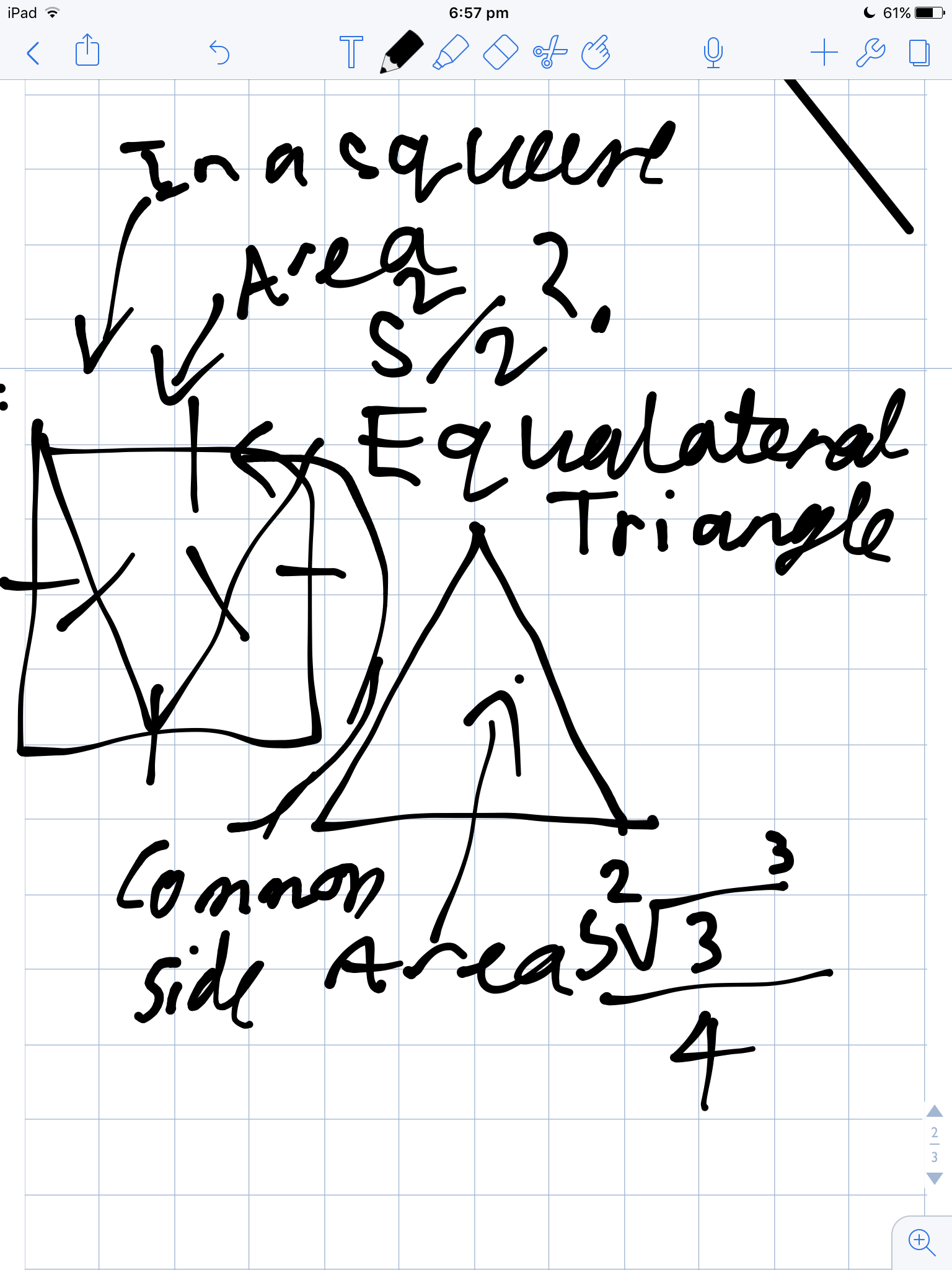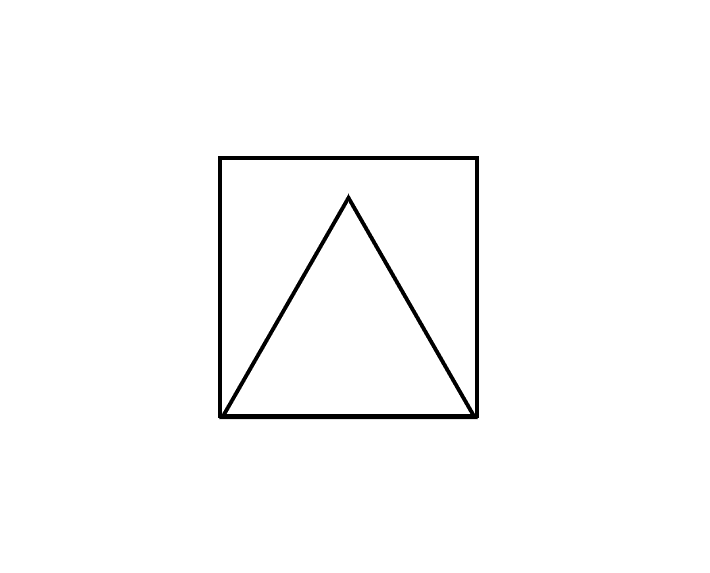# Equilateral Triangles

I found that the area of an equilateral triangle is sqrt(3)xS^2/4 where S is the side length But if I put it in a square, shouldn't the area be s^2/2?Note by Zoe Codrington
2 years, 9 months ago

This discussion board is a place to discuss our Daily Challenges and the math and science related to those challenges. Explanations are more than just a solution — they should explain the steps and thinking strategies that you used to obtain the solution. Comments should further the discussion of math and science.

When posting on Brilliant:

• Use the emojis to react to an explanation, whether you're congratulating a job well done , or just really confused .
• Ask specific questions about the challenge or the steps in somebody's explanation. Well-posed questions can add a lot to the discussion, but posting "I don't understand!" doesn't help anyone.
• Try to contribute something new to the discussion, whether it is an extension, generalization or other idea related to the challenge.

MarkdownAppears as
*italics* or _italics_ italics
**bold** or __bold__ bold
- bulleted- list
• bulleted
• list
1. numbered2. list
1. numbered
2. list
Note: you must add a full line of space before and after lists for them to show up correctly
paragraph 1paragraph 2

paragraph 1

paragraph 2

[example link](https://brilliant.org)example link
> This is a quote
This is a quote
    # I indented these lines
# 4 spaces, and now they show
# up as a code block.

print "hello world"
# I indented these lines
# 4 spaces, and now they show
# up as a code block.

print "hello world"
MathAppears as
Remember to wrap math in $$ ... $$ or $ ... $ to ensure proper formatting.
2 \times 3 $2 \times 3$
2^{34} $2^{34}$
a_{i-1} $a_{i-1}$
\frac{2}{3} $\frac{2}{3}$
\sqrt{2} $\sqrt{2}$
\sum_{i=1}^3 $\sum_{i=1}^3$
\sin \theta $\sin \theta$
\boxed{123} $\boxed{123}$

Sort by:

If you put it in a square, the top vertice won't touch the side of the square.

- 2 years, 9 months ago

Ok, thanks

- 2 years, 9 months ago

Did anyone else get this as their childhood interpretation of the A=bh/2 proof with equalateral triangles? I did.

- 2 years, 9 months ago

no, I better understood it with calculus. I am no geometer. I like to visualise it using functions and Cartesian graphs.

- 2 years, 8 months ago

It is impressive to do it with geometry; it requires a very visual brain.

- 2 years, 8 months ago

I know this is messy, but please?

- 2 years, 9 months ago- 2 years, 9 months ago

But is it possible to somehow prove without using Pythagoras Theorem or common sense? Edit:I just worked out the proof, but if you have ways to prove it other than the angles of an iscoceles triangle and possibly angles of an equaliteral triangle it would be interesting to hear.

- 2 years, 9 months ago